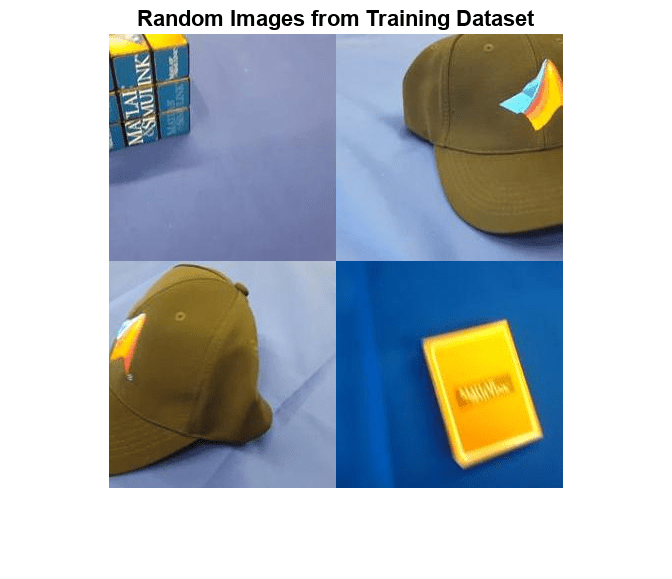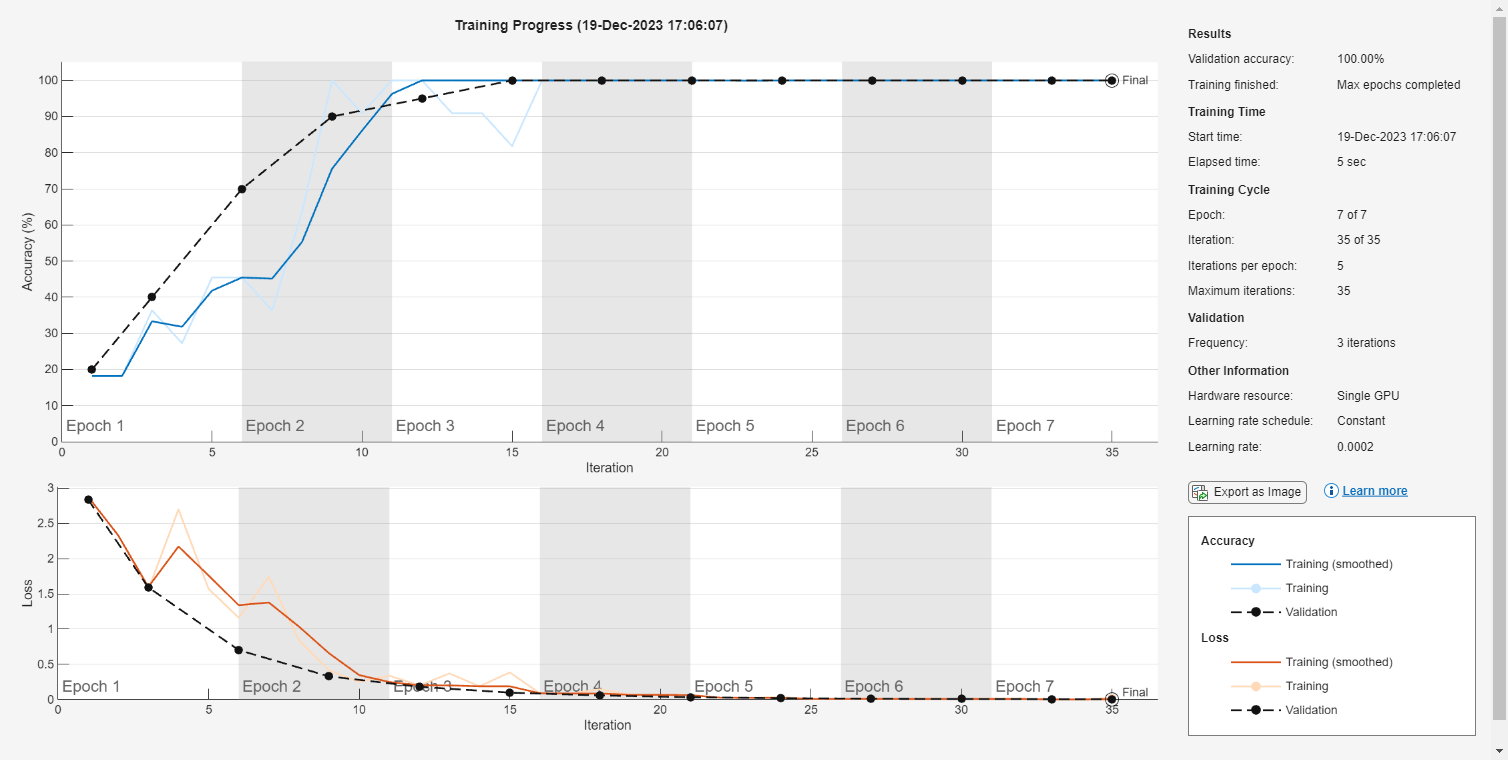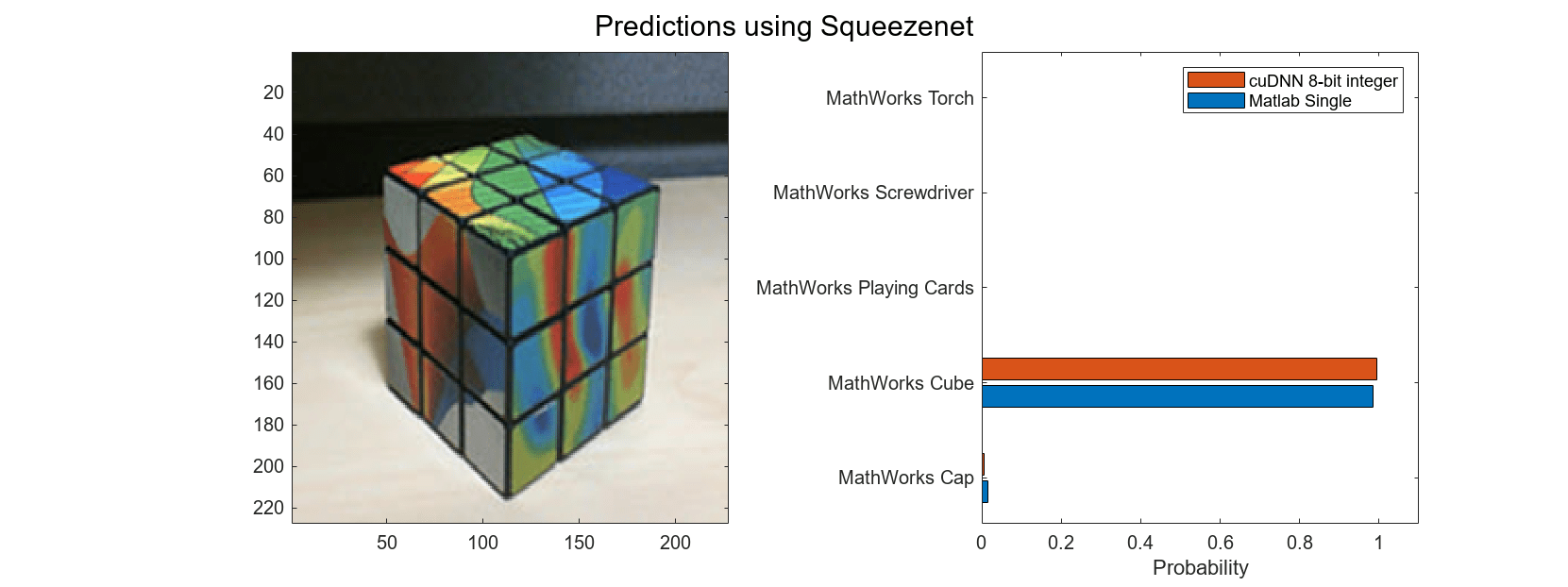## Generate `INT8` Code for Deep Learning Networks

Deep learning uses neural network architectures that contain many processing layers, including convolutional layers. Deep learning models typically work on large sets of labeled data. Training these models and performing inference is computationally intensive, consuming significant amount of memory. Neural networks use memory to store input data, parameters (weights), and activations from each layer as the input propagates through the network. The majority of the pretrained neural networks and neural networks trained by using Deep Learning Toolbox™ use single-precision floating point data types. Even networks that are small in size require a considerable amount of memory and hardware to perform these floating-point arithmetic operations. These restrictions can inhibit deployment of deep learning models to devices that have low computational power and smaller memory resources. By using a lower precision to store the weights and activations, you can reduce the memory requirements of the network.

You can use Deep Learning Toolbox in tandem with the Deep Learning Toolbox Model Quantization Library support package to reduce the memory footprint of a deep neural network by quantizing the weights, biases, and activations of convolution layers to 8-bit scaled integer data types. Then, you can use GPU Coder™ to generate CUDA® code for the optimized network. The generated code takes advantage of NVIDIA® CUDA deep neural network library (cuDNN) or the TensorRT™ high performance inference library. the generated code can be integrated into your project as source code, static or dynamic libraries, or executables that you can deploy to a variety of NVIDIA GPU platforms.

### Classify Images Using a Network Optimized for INT8 Inference

In this example, you use GPU Coder to generate optmized CUDA code for a deep convolutional neural network and classify an image. The generated code performs inference computation using 8-bit integer data type for the convolution layer. The example uses the pretrained `squeezenet` (Deep Learning Toolbox) convolutional neural network.

SqueezeNet has been trained on over a million images and can classify images into 1000 object categories (such as keyboard, coffee mug, pencil, and many animals). The network has learned rich feature representations for a wide range of images. The network takes an image as input and outputs a label for the object in the image together with the probabilities for each of the object categories.

This example consists of the following steps:

• Modify the `SqueezeNet` neural network to classify a smaller subset of images containing five object categories using transfer learning.

• Use the `calibrate` (Deep Learning Toolbox) function to exercise the network with sample inputs and collect range information to produce a calibration result file.

• Generate optimized code for the network by using the `codegen` command and the calibration result file.

Third-Party Prerequisites

Required

• CUDA enabled NVIDIA® GPU and compatible driver.

Optional

For non-MEX builds such as static, dynamic libraries or executables, this example has the following additional requirements.

Transfer Learning Using SqueezeNet

To perform classification on a new set of images, you fine-tune a pretrained SqueezeNet convolutional neural network by transfer learning. In transfer learning, you can take a pretrained network and use it as a starting point to learn a new task. Fine-tuning a network with transfer learning is usually much faster and easier than training a network with randomly initialized weights from scratch. You can quickly transfer learned features to a new task using a smaller number of training images.

Unzip and load the new images as an image datastore. The `imageDatastore` function automatically labels the images based on folder names and stores the data as an `ImageDatastore` object. An image datastore enables you to store large image data, including data that does not fit in memory, and efficiently read batches of images during training of a convolutional neural network. Divide the data into training and validation data sets. Use 70% of the images for training and 30% for validation. `splitEachLabel` splits the `imds` datastore into two new datastores.

```unzip('MerchData.zip'); imds = imageDatastore('MerchData', ... 'IncludeSubfolders',true, ... 'LabelSource','foldernames'); [imdsTrain,imdsValidation] = splitEachLabel(imds,0.7,'randomized'); numTrainImages = numel(imdsTrain.Labels); idx = randperm(numTrainImages,4); img = imtile(imds, 'Frames', idx); figure imshow(img) title('Random Images from Training Dataset');````net = squeezenet;`

The object `net` contains the `DAGNetwork` object. The first layer, the image input layer, requires input images of size 227-by-227-by-3, where 3 is the number of color channels. You can use the `analyzeNetwork` (Deep Learning Toolbox) function to display an interactive visualization of the network architecture, to detect errors and issues in the network, and to display detailed information about the network layers. The layer information includes the sizes of layer activations and learnable parameters, the total number of learnable parameters, and the sizes of state parameters of recurrent layers.

`inputSize = net.Layers(1).InputSize;`

Replace Final Layers

The convolutional layers of the network extract image features that the last learnable layer and the final classification layer use to classify the input image. These two layers, `'conv10'` and `'ClassificationLayer_predictions'` in SqueezeNet, contain information on how to combine the features that the network extracts into class probabilities, a loss value, and predicted labels.

To retrain a pretrained network to classify new images, replace these two layers with new layers adapted to the new data set. You can do this manually or use the helper function `findLayersToReplace` to find these layers automatically.

```lgraph = layerGraph(net); [learnableLayer,classLayer] = findLayersToReplace(lgraph); numClasses = numel(categories(imdsTrain.Labels)); newConvLayer = convolution2dLayer([1, 1],numClasses,'WeightLearnRateFactor',... 10,'BiasLearnRateFactor',10,"Name",'new_conv'); lgraph = replaceLayer(lgraph,'conv10',newConvLayer); newClassificatonLayer = classificationLayer('Name','new_classoutput'); lgraph = replaceLayer(lgraph,'ClassificationLayer_predictions',newClassificatonLayer);```

Train Network

The network requires input images of size 227-by-227-by-3, but the images in the image datastores have different sizes. Use an augmented image datastore to automatically resize the training images. Specify additional augmentation operations to perform on the training images: randomly flip the training images along the vertical axis, and randomly translate them up to 30 pixels horizontally and vertically. Data augmentation helps prevent the network from over-fitting and memorizing the exact details of the training images.

```pixelRange = [-30 30]; imageAugmenter = imageDataAugmenter( ... 'RandXReflection',true, ... 'RandXTranslation',pixelRange, ... 'RandYTranslation',pixelRange); augimdsTrain = augmentedImageDatastore(inputSize(1:2),imdsTrain, ... 'DataAugmentation',imageAugmenter);```

To automatically resize the validation images without performing further data augmentation, use an augmented image datastore without specifying any additional preprocessing operations.

`augimdsValidation = augmentedImageDatastore(inputSize(1:2),imdsValidation);`

Specify the training options. For transfer learning, keep the features from the early layers of the pretrained network (the transferred layer weights). To slow down learning in the transferred layers, set the initial learning rate to a small value. In the previous step, you increased the learning rate factors for the convolutional layer to speed up learning in the new final layers. This combination of learning rate settings results in fast learning only in the new layers and slower learning in the other layers. When performing transfer learning, you do not need to train for as many epochs. An epoch is a full training cycle on the entire training data set. Specify the mini-batch size to be 11 so that in each epoch you consider all of the data. The software validates the network every `ValidationFrequency` iterations during training.

```options = trainingOptions('sgdm', ... 'MiniBatchSize',11, ... 'MaxEpochs',7, ... 'InitialLearnRate',2e-4, ... 'Shuffle','every-epoch', ... 'ValidationData',augimdsValidation, ... 'ValidationFrequency',3, ... 'Verbose',false, ... 'Plots','training-progress');```

Train the network that consists of the transferred and new layers.

`netTransfer = trainNetwork(augimdsTrain,lgraph,options);````classNames = netTransfer.Layers(end).Classes; save('mySqueezenet.mat','netTransfer');```

Generate Calibration Result File for the Network

Create a `dlquantizer` object and specify the network. Code generation does not support quantized deep neural networks produced by the `quantize` (Deep Learning Toolbox) function.

`quantObj = dlquantizer(netTransfer);`

Define a metric function to use to compare the behavior of the network before and after quantization.

`type('hComputeModelAccuracy.m');`
```function accuracy = hComputeModelAccuracy(predictionScores, net, dataStore) %% Computes model-level accuracy statistics % Load ground truth tmp = readall(dataStore); groundTruth = tmp.response; % Compare with predicted label with actual ground truth predictionError = {}; for idx=1:numel(groundTruth) [~, idy] = max(predictionScores(idx,:)); yActual = net.Layers(end).Classes(idy); predictionError{end+1} = (yActual == groundTruth(idx)); %#ok end % Sum all prediction errors. predictionError = [predictionError{:}]; accuracy = sum(predictionError)/numel(predictionError); end ```

Specify the metric function in a `dlquantizationOptions` object.

```quantOpts = dlquantizationOptions('MetricFcn', ... {@(x)hComputeModelAccuracy(x,netTransfer,augimdsValidation)});```

Use the `calibrate` function to exercise the network with sample inputs and collect range information. The `calibrate` function exercises the network and collects the dynamic ranges of the weights and biases in the convolution and fully connected layers of the network and the dynamic ranges of the activations in all layers of the network. The function returns a table. Each row of the table contains range information for a learnable parameter of the optimized network.

```calResults = calibrate(quantObj,augimdsTrain); save('squeezenetCalResults.mat','calResults'); save('squeezenetQuantObj.mat','quantObj');```

You can use the `validate` function to quantize the learnable parameters in the convolution layers of the network and exercise the network. The function uses the metric function defined in the `dlquantizationOptions` object to compare the results of the network before and after quantization.

`valResults = validate(quantObj,augimdsValidation,quantOpts);`

Create an Entry-Point Function

Write an entry-point function in MATLAB that:

• Uses the `coder.loadDeepLearningNetwork` function to load a deep learning model and to construct and set up a CNN class. For more information, see Load Pretrained Networks for Code Generation.

• Calls the `predict` function to predict the responses.

`type('predict_int8.m');`
```function out = predict_int8(netFile, in) persistent mynet; if isempty(mynet) mynet = coder.loadDeepLearningNetwork(netFile); end out = predict(mynet,in); end ```

A persistent object `mynet` loads the `DAGNetwork` object. At the first call to the entry-point function, the persistent object is constructed and set up. On subsequent calls to the function, the same object is reused to call `predict` on inputs, avoiding reconstructing and reloading the network object.

Note

Ensure that all the preprocessing operations performed in the calibration and validation steps are included in the design file.

Code Generation by Using `codegen`

To configure build settings such as output file name, location, and type, you create coder configuration objects. To create the objects, use the `coder.gpuConfig` function. For example, when generating CUDA MEX using the `codegen` command, use `cfg = coder.gpuConfig('mex');`

To specify code generation parameters for cuDNN, set the `DeepLearningConfig` property to a `coder.CuDNNConfig` object that you create by using `coder.DeepLearningConfig`.

```cfg = coder.gpuConfig('mex'); cfg.TargetLang = 'C++'; cfg.GpuConfig.ComputeCapability = '6.1'; cfg.DeepLearningConfig = coder.DeepLearningConfig('cudnn'); cfg.DeepLearningConfig.AutoTuning = true; cfg.DeepLearningConfig.CalibrationResultFile = 'squeezenetQuantObj.mat'; cfg.DeepLearningConfig.DataType = 'int8';```

Specify the location of the MAT-file containing the calibration data.

Specify the precision of the inference computations in supported layers by using the `DataType` property. For 8-bit integer, use `'int8'`. Use the `ComputeCapability` property of the code configuration object to set the appropriate compute capability value.

Run the `codegen` command. The `codegen` command generates CUDA code from the `predict_int8.m` MATLAB entry-point function.

```inputs = {coder.Constant('mySqueezenet.mat'),ones(inputSize,'uint8')}; codegen -config cfg -args inputs predict_int8```
```Code generation successful. ```

When code generation is successful, you can view the resulting code generation report by clicking View Report in the MATLAB Command Window. The report is displayed in the Report Viewer window. If the code generator detects errors or warnings during code generation, the report describes the issues and provides links to the problematic MATLAB code.

Run the Generated MEX

The image that you want to classify must have the same size as the input size of the network. Read the image that you want to classify and resize it to the input size of the network. This resizing slightly changes the aspect ratio of the image.

```testImage = imread("MerchDataTest.jpg"); testImage = imresize(testImage,inputSize(1:2));```

Call SqueezeNet predict on the input image.

```predictScores(:,1) = predict(netTransfer,testImage)'; predictScores(:,2) = predict_int8_mex('mySqueezenet.mat',testImage);```

Display the predicted labels and their associated probabilities as a histogram.

```h = figure; h.Position(3) = 2*h.Position(3); ax1 = subplot(1,2,1); ax2 = subplot(1,2,2); image(ax1,testImage); barh(ax2,predictScores) xlabel(ax2,'Probability') yticklabels(ax2,classNames) ax2.XLim = [0 1.1]; ax2.YAxisLocation = 'left'; legend('Matlab Single','cuDNN 8-bit integer'); sgtitle('Predictions using Squeezenet')```Helper Functions

```function [learnableLayer,classLayer] = findLayersToReplace(lgraph) % findLayersToReplace(lgraph) finds the single classification layer and the % preceding learnable (fully connected or convolutional) layer of the layer % graph lgraph. if ~isa(lgraph,'nnet.cnn.LayerGraph') error('Argument must be a LayerGraph object.') end % Get source, destination, and layer names. src = string(lgraph.Connections.Source); dst = string(lgraph.Connections.Destination); layerNames = string({lgraph.Layers.Name}'); % Find the classification layer. The layer graph must have a single % classification layer. isClassificationLayer = arrayfun(@(l) ... (isa(l,'nnet.cnn.layer.ClassificationOutputLayer') ... |isa(l,'nnet.layer.ClassificationLayer')), ... lgraph.Layers); if sum(isClassificationLayer) ~= 1 error('Layer graph must have a single classification layer.') end classLayer = lgraph.Layers(isClassificationLayer); % Traverse the layer graph in reverse starting from the classification % layer. If the network branches, throw an error. currentLayerIdx = find(isClassificationLayer); while true if numel(currentLayerIdx) ~= 1 msg = ['Layer graph must have a single learnable layer ' ... 'preceding the classification layer.']; error(msg) end currentLayerType = class(lgraph.Layers(currentLayerIdx)); isLearnableLayer = ismember(currentLayerType, ... ['nnet.cnn.layer.FullyConnectedLayer','nnet.cnn.layer.Convolution2DLayer']); if isLearnableLayer learnableLayer = lgraph.Layers(currentLayerIdx); return end currentDstIdx = find(layerNames(currentLayerIdx) == dst); currentLayerIdx = find(src(currentDstIdx) == layerNames); end end```

### Limitations

• When performing inference in `INT8` precision using cuDNN version 8.1.0, issues in the NVIDIA library may cause significant degradation in performance.

• The following layers are not supported for 8-bit integer quantization when targeting the NVIDIA CUDA deep neural network library (cuDNN) library.

• `leakyReluLayer`

• `clippedReluLayer`

• `globalAveragePooling2dLayer`